Next: Axisymmetric Irrotational Flow in Up: Axisymmetric Incompressible Inviscid Flow Previous: Stokes Stream Function

# Axisymmetric Velocity Fields

According to the analysis of Appendix C, Equations (7.3) and (7.4) imply that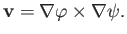(7.5)

When the fluid velocity is written in this form it becomes obvious that the incompressibility constraint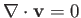is satisfied [because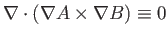--see Equations (A.175) and (A.176)]. It is also clear that the Stokes stream function,, is undefined to an arbitrary additive constant.

In fact, the most general expression for an axisymmetric incompressible flow pattern is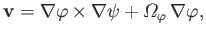(7.6)

where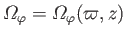is the angular velocity of flow circulating about the-axis. (This follows because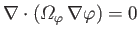when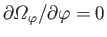. See Appendix C.) The previous expression implies that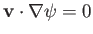(because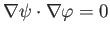when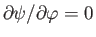). In other words, when plotted in the meridian plane, streamlines in a general axisymmetric flow pattern correspond to contours of.

Making use of the vector identities (A.176) and (A.178), we can also write Equation (7.6) in the form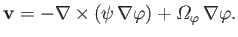(7.7)

It follows from the identity (A.177) that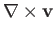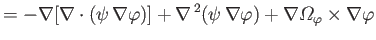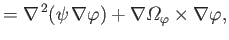(7.8)

because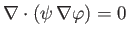, by symmetry. Hence, the vorticity of a general axisymmetric flow pattern is written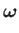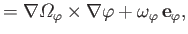(7.9)

where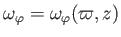, and [see Equations (C.52)-(C.54)]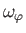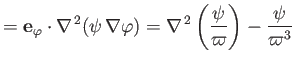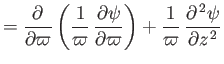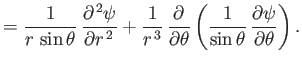(7.10)

In the following, we shall concentrate on axisymmetric flow patterns in which there is no circulation about the-axis (i.e.,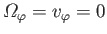).Next: Axisymmetric Irrotational Flow in Up: Axisymmetric Incompressible Inviscid Flow Previous: Stokes Stream Function
Richard Fitzpatrick 2016-03-31